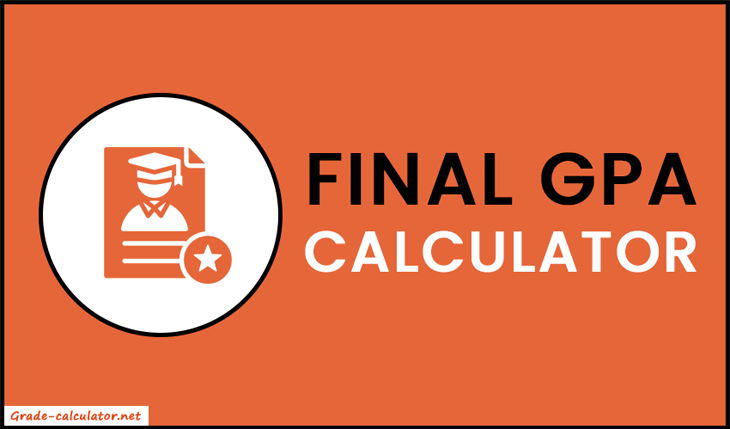# Final GPA Calculator

Use our Final GPA Calculator to find what additional GPA is needed in your next term in order to reach your target GPA.

Welcome to our Final GPA Calculator. It allows you to calculate the additional GPA needed to get the desired GPA in your final exam. It takes your current GPA, target GPA, current credits, and additional credits as input and gives what additional GPA is needed to achieve the desired GPA. In short, it's the best tool to set your goals and plan the course schedule accordingly.### How to Calculate Final GPA?

To calculate the final GPA, we will use the following formula.

Final GPA = ((CHours + AHours) × TGPA) - (CHours × CGPA) / AHours

Where,
CHours = Current Credit Hours
TGPA = Target GPA
CGPA = Current GPA

Now let's take an example.

#### Example:

Calculate the final GPA for the following values:

• Current GPA = 3.23
• Target GPA = 3.5
• Current Credit Hours = 25
• Additional Credit Hours = 30
##### Solution:

Place all the given values in the formula and we get:

Final GPA = ((25+30) × 3.5) - (25 × 3.23) / 30

= (192.5 - 80.75) / 30

= 111.75 / 30